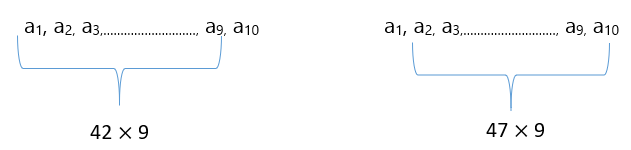# CAT 2020 Question Paper | Quants Slot 2

###### CAT Previous Year Paper | CAT Quants Questions | Question 3

If we analyze CAT previous year paper over time, we see that the major chunk of the Quantitative Aptitude section comprises of questions from Arithmetic. Averages forms one of the topics under Arithmetic and hence is crucial for your CAT preparation online. The best way to get a hang of tricky questions on simpler topics like Averages is by practicing CAT previous year paper. Here is a beautiful question from the topic to get you going. Solve this question from CAT Question paper 2020 and see if you get the correct answer without going through the solutions provided.

Question 3 : In a group of 10 students, the mean of the lowest 9 scores is 42 while the mean of the highest 9 scores is 47. For the entire group of 10 students, the maximum possible mean exceeds the minimum possible mean by

1. 5
2. 3
3. 4
4. 6

## Best CAT Coaching in Chennai

#### CAT Coaching in Chennai - CAT 2022Limited Seats Available - Register Now!Here a2 to a9 is common to both the terms
So, a1 + (a2 to a9) = 42 × 9
a10 + (a2 to a9) = 47 × 9
Solving these two a10 - a1 = 45
a1, a2, a3,………………………, a9, (a1 + 45)
One instance is every number is 42
42, 42, …………………, 42, 42+45 (a1 to a9 are equal) -----------(1)
Another instance is every number is 47
47 – 45, 47, …………………, 47, 47 (a2 to a10 are equal) ---------(2)
Mean of (1) = 46.5
Mean of (2) = 42.5
(1) – (2) = 4

###### CAT Coaching in ChennaiCAT 2023

Classroom Batches Starting Now! @Gopalapuram

###### Best CAT Coaching in Chennai Introductory offer of 5000/-

Attend a Demo Class

##### Where is 2IIM located?

2IIM Online CAT Coaching
A Fermat Education Initiative,
58/16, Indira Gandhi Street,
Kaveri Rangan Nagar, Saligramam, Chennai 600 093

##### How to reach 2IIM?

Mobile: (91) 99626 48484 / 94459 38484
WhatsApp: WhatsApp Now
Email: info@2iim.com